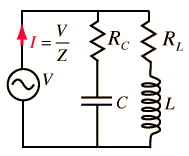# Parallel RLC Circuit

Quick Question...

Given:Can I still use the general impedance equation for parallel RLC circuits even though the resistors are not in parallel with the other components? Substituting the two resistors for a single resistor with the equivalency resistance?

The first leg is (Rc and C in series) and this combination is in parallel with (Rl and L in series)

The first leg is (Rc and C in series) and this combination is in parallel with (Rl and L in series)

Does this give us...

1) Q2/C - ICRC - V = 0
2) ILRL - (L * diL/dt) - V = 0

Or am I just completely off?

The circuit is an AC circuit so you need to use Reactance of C..... Xc =1/ωC to get an expression for the impedance of the Rc C arm.
You need to use Xl = ωL to get an expression for the Impedance of the Rl L arm
The overall impedance is these 2 impedances in parallel.